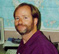## Phase and Gain alignmentStarted by 6 years ago7 replieslatest reply 6 years ago200 views

Hi all

I have simultaneously recorded data coming from three pre-amplifiers with same input applied to these pre-amps. After post analysis, i found that there are phase and gain differences among these outputs ( which should be zero in ideal case). I set one preamplifer output as reference and measure the phase and gain difference values of other two preamps with respect to this reference using "fft technique" for a typical frequency value.

Now question is how can i use these phase/gain difference values to align all three signals in software?

Note: Idea is to save these phase/gain coefficients permanently and multiply them with each corresponding preamplifier's outputs (excluding reference ) sample by sample for each acquisition so that inherent phase/gain difference b/w preamplifiers can be adjusted without modifying the electronics.

Thanks

[ - ]You seem to be describing an equalizer.   If you can use one of the amps output as the reference, then just generate the error signal between the reference and the amp being equalized and use that error to train the equalizer.

If you just want to do it once, train the equalizer with an appropriate test signal until the coefficients stabilize, then freeze the coefficients and just use that as the equalizing filter.

[ - ]Thanks for reply. I am trying to calibrate electronics of linear array having three sensors. Only if calibrated properly, i will be able to find correct DOA of signals impinging on this array. Also, phase/gain difference is frequency dependent.

[ - ]I suggest you calibrate by applying frequency sweep covering your signal range then do fft on input and each of three outputs. Each of three fft ratios of output to input is the system response.

Alternatively assume output1 is your reference then do fft ratio of output2 relative to 1 and 3 relative to 1.

then do ifft of ratios and get your coeffs at suitable resolution. Apply them on actual signals when system is running.

[ - ]Just calibrating the amplifiers won't be enough.  The sensors will need to be calibrated also.  I used to work with DOA of acoustic signals.

We calibrated the mics (and amplifiers) with a tube with a speaker at one end and absorbing foam on the sides.  The idea was to have the signal act like a plane wave.  We fed the speaker with pseudorandom noise, but a frequency sweep would also work.

[ - ]Thanks for reply. Your work seems quite close to what i want to do (calibration including sensors+preamps was next step for me). Can you kindly explain  did you do this calibration (i.e. phase and gain adjustment as i understood) in hardware part or software part? My requirement is to do this in software but how to do this is a question.

Thanks

[ - ]